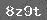# 小学数学第三册期中试卷

一、 判断题(每道小题 4 8 )

1. (1)1×1=1÷1　　　　　　　　　　　　　　　　　　　　(　　　 )

(2)1米等于100厘米．　　　　　　　　　　　　　　　　　(　　　 )

2. (1)36连加是多少, 列式是3×6　　　　　　　　　　(　　　 )

(2)8分成2, 每份是4　　　　　　　　　　　　　　 (　　　 )

1.2. 填单位名称．

3. 在○里填上+-、×、÷．

11=0

11=1

11=2

4. 在○里填上+-、×、÷．

93=3

4=84

55=25

5. 4×5=(　　　 )　　　 (　　　 )×4=16

(　　　 )×1=1　　　 3×(　　　 )=15

6. (1)6×5=(　　　 )表示(　　　 )(　　　 )连加．

(2)18÷3=(　　　 )表示把(　　　 )平均分成(　　　 ), 每份是(　　　 ), 还表示(　　　 )里面有(　　　 )(　　　 )

7. 看图填空．(　　　 )×(　　　 )=(　　　 )

(　　　 )÷(　　　 )=(　　　 )

(　　　 )÷(　　　 )=(　　　 )

8. 按要求把算式填在圆圈里．

2×2　　　　　　　 12÷2　　　　　 2+2

18÷3　　　　　　 16÷4　　　　　 24÷4

20÷4　　　　　　 15÷3　　　　　 5×11. 　　75-50=

24+9+8=

30-19+10=

50-8+20=

72-(10+30)=

15+9-6=

2. 　 6×6=

12÷4=

4×3=

6÷3=

1×5=

21-7=

3. 　25÷5=

6×8=

12÷6=

8+38=

20÷4=

24+4=

1. 63的和是多少?

2. 被乘数是4,乘数是6,积是多少?

3. 30里面有几个6?

4. 5乘以2得多少?

1. 有两盘梨, 一盘5, 另一盘4, 一共有多少个?

2. 商店里有15辆自行车，又运来18辆，卖出了20辆，一共有多少辆？还剩多少辆？

3. 学校有3行柿子树,每行5,一共有柿子树多少棵?

4. 一个练习本2角钱,4个同样的练习本多少钱?

### 相关推荐

 留言与评论（共有 0 条评论）

 验证码：